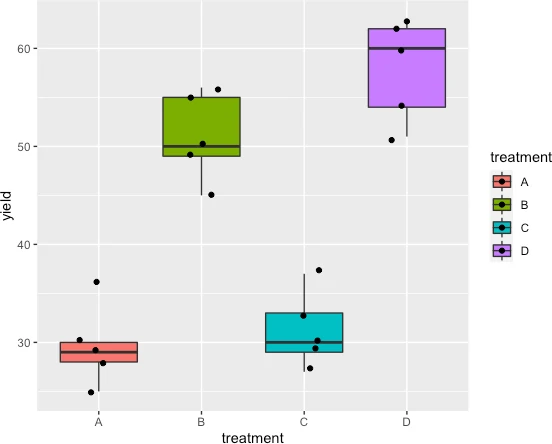# Brown-Forsythe test for equality of variances in R## What is the Brown-Forsythe test?

• Brown-Forsythe test is used for testing the equality variances (homogeneity of variances) among different groups. It is a robust test when the underlying data does not meet assumptions of normality. Brown-Forsythe test is proposed by Morton Brown and Alan Forsythe in 1974.
• Both Levene’s test and Brown-Forsythe test can be used for testing for homogeneity of variances on nonnormal data. But, the Brown-Forsythe test is more robust than Levene’s test when data distributions are skewed or heavy-tailed (Cauchy distribution). Brown-Forsythe test is a modified version of Levene’s test.
• Levene’s test s based on group means whereas the Brown-Forsythe test is based on group medians or trimmed means.

## Brown-Forsythe test hypothesis

• Null hypothesis (H0): Group variances are equal (σ1222=…=σk2)
• Alternative hypothesis (Ha): At least one group variance is not equal (σi2≠σj2)

## Calculate Brown-Forsythe test in R

We will use the tidyverse and vGWAS R packages for this tutorial.

### Get dataset

Suppose we have four treatment groups (A. B, C, and D) and their plant yield. We would like to check if the variances among the four treatment groups are equal or not. The Homogeneity of variances needs to be checked before performing the ANOVA analysis.

# R version 4.1.2 (2021-11-01)
library(tidyverse)
# output
treatment yield
<chr>     <dbl>
1 A            25
2 A            30


### Get variance and visualize the data spread (boxplot)

Calculate variance for each treatment group,

df %>% group_by(treatment) %>% summarise(var = var(yield))

# output
treatment   var
<chr>     <dbl>
1 A          16.3
2 B          20.5
3 C          15.2
4 D          27.5


Generate a boxplot to see the data distribution by treatments. Using boxplot, we can easily detect how data is spread for each treatment,

ggplot(df, aes(x = treatment, y = yield, fill = treatment)) +
geom_boxplot(outlier.shape = NA) + geom_jitter(width = 0.2)### Perform Brown-Forsythe test for equal variance

We will use the brown.forsythe.test function from vGWAS package to perform the Brown-Forsythe test for equal variance

Pass the following parameters to brown.forsythe.test function,

• y : a numeric vector of the dependent variable
• group : Groups (independent variable)
library(vGWAS)
brown.forsythe.test(y = df$yield, group = df$treatment)

# output

Brown-Forsythe test based on the absolute deviations from the
median

data:  df\$yield
Test Statistic = 0.22663, p-value = 0.8765


The Brown-Forsythe test results indicate that variances are not significantly different [W = 0.22, p = 0.87] among the groups. Hence, we fail to reject the null hypothesis (as p > 0.05) that group variances are equal. As the group variances are not different, the Homogeneity of variance assumption for the one-way ANOVA test is valid.

Note: Brown-Forsythe test for equality of variances is different than the Brown-Forsythe test for equality of means. The later test can be performed using the bf.test function from the onewaytests package.

## Enhance your skills with statistical courses using R

If you have any questions, comments, corrections, or recommendations, please email me at reneshbe@gmail.com